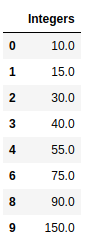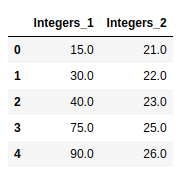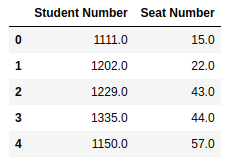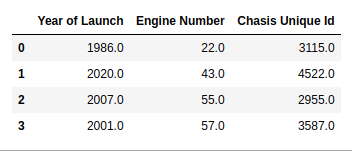# How to Drop Rows with NaN Values in Pandas DataFrame?

NaN stands for Not A Number and is one of the common ways to represent the missing value in the data. It is a special floating-point value and cannot be converted to any other type than float. NaN value is one of the major problems in Data Analysis. It is very essential to deal with NaN in order to get the desired results. In this article, we will discuss how to drop rows with NaN values.

We can drop Rows having NaN Values in Pandas DataFrame by using dropna() function

` df.dropna() `

It is also possible to drop rows with NaN values with regard to particular columns using the following statement:

`df.dropna(subset, inplace=True)`

With `inplace` set to `True` and subset set to a list of column names to drop all rows with NaN under those columns.

Example 1:

 `# importing libraries ` `import` `pandas as pd ` `import` `numpy as np ` ` `  `num ``=` `{``'Integers'``: [``10``, ``15``, ``30``, ``40``, ``55``, np.nan, ` `                    ``75``, np.nan, ``90``, ``150``, np.nan]} ` ` `  `# Create the dataframe ` `df ``=` `pd.DataFrame(num, columns ``=``[``'Integers'``]) ` ` `  `# dropping the rows having NaN values ` `df ``=` `df.dropna() ` ` `  `# printing the result ` `df `

Output:Note: We can also reset the indices using the method reset_index()

`df = df.reset_index(drop=True)`

Example 2:

 `# importing libraries  ` `import` `pandas as pd ` `import` `numpy as np ` ` `  `nums ``=` `{``'Integers_1'``: [``10``, ``15``, ``30``, ``40``, ``55``, np.nan,  ` `                       ``75``, np.nan, ``90``, ``150``, np.nan], ` `           ``'Integers_2'``: [np.nan, ``21``, ``22``, ``23``, np.nan, ` `                          ``24``, ``25``, np.nan, ``26``, np.nan,  ` `                          ``np.nan]} ` ` `  `# Create the dataframe ` `df ``=` `pd.DataFrame(nums, columns ``=``[``'Integers_1'``, ``'Integers_2'``]) ` ` `  `# dropping the rows having NaN values ` `df ``=` `df.dropna() ` ` `  `# To reset the indices  ` `df ``=` `df.reset_index(drop ``=` `True``) ` ` `  `# Print the dataframe ` `df `

Output:Example 3:

 `# importing libraries  ` `import` `pandas as pd ` `import` `numpy as np ` ` `  `nums ``=` `{``'Student Number'``: [ ``1001``, ``1111``, ``1202``, ``1229``, ``1330``, ` `                           ``1335``, np.nan, ``1400``, ``1150``, np.nan], ` `           ``'Seat Number'``: [np.nan, ``15``, ``22``, ``43``, np.nan, ``44``, ` `                           ``55``, np.nan, ``57``, np.nan]} ` ` `  `# Create the dataframe ` `df ``=` `pd.DataFrame(nums, columns ``=``[``'Student Number'``, ``'Seat Number'``]) ` ` `  `# dropping the rows having NaN values ` `df ``=` `df.dropna() ` ` `  `# To reset the indices  ` `df ``=` `df.reset_index(drop ``=` `True``) ` ` `  `# Print the dataframe ` `df `

Output:Example 4:

 `# importing libraries  ` `import` `pandas as pd ` `import` `numpy as np ` ` `  `car ``=` `{``'Year of Launch'``: [ ``1999``, np.nan, ``1986``, ``2020``, np.nan, ` `                          ``1991``, ``2007``, ``2011``, ``2001``, ``2017``], ` `           ``'Engine Number'``: [np.nan, ``15``, ``22``, ``43``, ``44``, np.nan, ` `                             ``55``, np.nan, ``57``, np.nan],  ` `        ``'Chasis Unique Id'``: [``4023``, np.nan, ``3115``, ``4522``, ``3643``, ` `                             ``3774``, ``2955``, np.nan, ``3587``, np.nan]} ` ` `  `# Create the dataframe ` `df ``=` `pd.DataFrame(car, columns ``=``[``'Year of Launch'``, ``'Engine Number'``, ` `                                 ``'Chasis Unique Id'``]) ` ` `  `# dropping the rows having NaN values ` `df ``=` `df.dropna() ` ` `  `# To reset the indices  ` `df ``=` `df.reset_index(drop ``=` `True``) ` ` `  `# Print the dataframe ` `df `

Output:My Personal Notes arrow_drop_upIf you like GeeksforGeeks and would like to contribute, you can also write an article using contribute.geeksforgeeks.org or mail your article to contribute@geeksforgeeks.org. See your article appearing on the GeeksforGeeks main page and help other Geeks.

Please Improve this article if you find anything incorrect by clicking on the "Improve Article" button below.

Article Tags :

8

Please write to us at contribute@geeksforgeeks.org to report any issue with the above content.